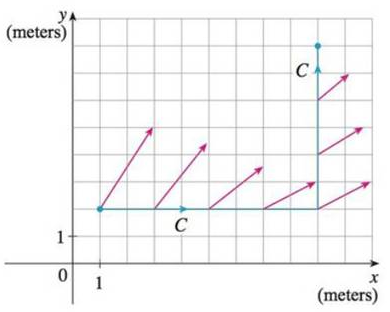Chapter 16.2, Problem 51E

Chapter
Section
Textbook Problem

An object moves along the curve C shown in the figure from (1, 2) to (9, 8). The lengths of the vectors in the force field F are measured in newtons by the scales on the axes. Estimate the work done by F on the object.To determine

To Estimate: The work done by the force field F on the object.

Explanation

Given data:

Refer to the Figure at the bottom the question 51 in the textbook.

An object moves along the curve C in the figure from the point (1,2) to (9,8) .

Formula used:

Write the expression to find work done by the object in moving the particle.

CFTds=i=1n[F(xi,yi,zi)T(xi,yi,zi)]Δsi (1)

Here,

T(x,y,z) is the unit tangent vector at the point (x,y,z) on the curve C ,

Δsi is the length between two points of each segment, and

n is the number of segments, where 7 segments from the figure.

From the Figure in the textbook, the length between any two points of each segment is 2.

Δsi=2

As the curve C is composed of straight line segments, FT is the scalar projection of force field vector onto the curve C.

Calculation of work done by the object:

Substitute 2 for Δsi in equation (1) and rewrite the expression as follows.

CFTds=i=17[F(xi,yi,zi)T(xi,yi,zi)]Δsi

CFTds=[F(x1,y1,z1)T(x1,y1,z1)+F(x2,y2,z2)T(x2,y2,z2)+F(x3,y3,z3)T(x3,y3,z3)+F(x4,y4,z4)T(x4,y4,z4)+F(x5,y5,z5)T(x5,y5,z

Still sussing out bartleby?

Check out a sample textbook solution.

See a sample solution

The Solution to Your Study Problems

Bartleby provides explanations to thousands of textbook problems written by our experts, many with advanced degrees!

Get Started

Find the 1000th derivative of f(x) = xex.

Single Variable Calculus: Early Transcendentals, Volume I

Solve the equations in Exercises 112 for x (mentally, if possible). 34x+1=0

Finite Mathematics and Applied Calculus (MindTap Course List)

The x-coordinate of the center of mass of the region bounded by , x = 1, x = 2, y = 0 is: ln 2 1 2 ln 2

Study Guide for Stewart's Single Variable Calculus: Early Transcendentals, 8th